# Inpainting using Sparse Regularization¶

Important: Please read the installation page for details about how to install the toolboxes. $\newcommand{\dotp}{\langle #1, #2 \rangle}$ $\newcommand{\enscond}{\lbrace #1, #2 \rbrace}$ $\newcommand{\pd}{ \frac{ \partial #1}{\partial #2} }$ $\newcommand{\umin}{\underset{#1}{\min}\;}$ $\newcommand{\umax}{\underset{#1}{\max}\;}$ $\newcommand{\umin}{\underset{#1}{\min}\;}$ $\newcommand{\uargmin}{\underset{#1}{argmin}\;}$ $\newcommand{\norm}{\|#1\|}$ $\newcommand{\abs}{\left|#1\right|}$ $\newcommand{\choice}{ \left\{ \begin{array}{l} #1 \end{array} \right. }$ $\newcommand{\pa}{\left(#1\right)}$ $\newcommand{\diag}{{diag}\left( #1 \right)}$ $\newcommand{\qandq}{\quad\text{and}\quad}$ $\newcommand{\qwhereq}{\quad\text{where}\quad}$ $\newcommand{\qifq}{ \quad \text{if} \quad }$ $\newcommand{\qarrq}{ \quad \Longrightarrow \quad }$ $\newcommand{\ZZ}{\mathbb{Z}}$ $\newcommand{\CC}{\mathbb{C}}$ $\newcommand{\RR}{\mathbb{R}}$ $\newcommand{\EE}{\mathbb{E}}$ $\newcommand{\Zz}{\mathcal{Z}}$ $\newcommand{\Ww}{\mathcal{W}}$ $\newcommand{\Vv}{\mathcal{V}}$ $\newcommand{\Nn}{\mathcal{N}}$ $\newcommand{\NN}{\mathcal{N}}$ $\newcommand{\Hh}{\mathcal{H}}$ $\newcommand{\Bb}{\mathcal{B}}$ $\newcommand{\Ee}{\mathcal{E}}$ $\newcommand{\Cc}{\mathcal{C}}$ $\newcommand{\Gg}{\mathcal{G}}$ $\newcommand{\Ss}{\mathcal{S}}$ $\newcommand{\Pp}{\mathcal{P}}$ $\newcommand{\Ff}{\mathcal{F}}$ $\newcommand{\Xx}{\mathcal{X}}$ $\newcommand{\Mm}{\mathcal{M}}$ $\newcommand{\Ii}{\mathcal{I}}$ $\newcommand{\Dd}{\mathcal{D}}$ $\newcommand{\Ll}{\mathcal{L}}$ $\newcommand{\Tt}{\mathcal{T}}$ $\newcommand{\si}{\sigma}$ $\newcommand{\al}{\alpha}$ $\newcommand{\la}{\lambda}$ $\newcommand{\ga}{\gamma}$ $\newcommand{\Ga}{\Gamma}$ $\newcommand{\La}{\Lambda}$ $\newcommand{\si}{\sigma}$ $\newcommand{\Si}{\Sigma}$ $\newcommand{\be}{\beta}$ $\newcommand{\de}{\delta}$ $\newcommand{\De}{\Delta}$ $\newcommand{\phi}{\varphi}$ $\newcommand{\th}{\theta}$ $\newcommand{\om}{\omega}$ $\newcommand{\Om}{\Omega}$

This numerical tour explores the use of sparse energies to regularize the image inpaiting problem.

In :
from __future__ import division

import numpy as np
import scipy as scp
import pylab as pyl
import matplotlib.pyplot as plt

from nt_toolbox.general import *
from nt_toolbox.signal import *

import warnings
warnings.filterwarnings('ignore')

%matplotlib inline


Here we consider inpainting of damaged observation without noise.

## Sparse Regularization¶

This tour consider measurements $y=\Phi f_0 + w$ where $\Phi$ is a masking operator and $w$ is an additive noise.

This tour is focused on using sparsity to recover an image from the measurements $y$. It considers a synthesis-based regularization, that compute a sparse set of coefficients $(a_m^{\star})_m$ in a frame $\Psi = (\psi_m)_m$ that solves $$a^{\star} \in \text{argmin}_a \: \frac{1}{2}\|y-\Phi \Psi a\|^2 + \lambda J(a)$$

where $\lambda$ should be adapted to the noise level $\|w\|$. Since in this tour we consider damaged observation without noise, i.e. $w=0$, we use either a very small value of $\lambda$, or we decay its value through the iterations of the recovery process.

Here we use the notation $$\Psi a = \sum_m a_m \psi_m$$ to indicate the reconstruction operator, and $J(a)$ is the $\ell^1$ sparsity prior $$J(a)=\sum_m \|a_m\|.$$

## Missing Pixels and Inpainting¶

Inpainting corresponds to filling holes in images. This corresponds to a linear ill posed inverse problem.

You might want to do first the numerical tour Variational image inpaiting that use Sobolev and TV priors to performs the inpainting.

First we load the image to be inpainted.

In :
n = 128
f0 = rescale(f0[256-n//2:256+n//2,256-n//2:256+n//2])


Display it.

In :
plt.figure(figsize = (6,6))
imageplot(f0, 'Image f_0')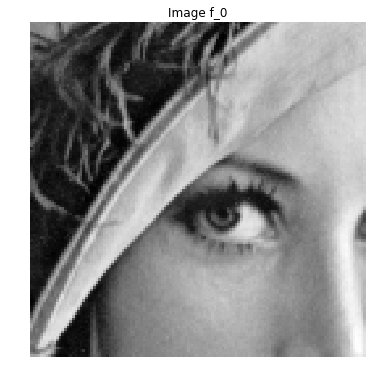Amount of removed pixels.

In :
rho = .7


Then we construct a mask $\Omega$ made of random pixel locations.

In :
from numpy import random

Omega = np.zeros([n, n])
sel = random.permutation(n**2)
np.ravel(Omega)[sel[np.arange(int(rho*n**2))]] = 1


The damaging operator put to zeros the pixel locations $x$ for which $\Omega(x)=1$

In :
Phi = lambda f, Omega: f*(1-Omega)


The damaged observations reads $y = \Phi f_0$.

In :
y = Phi(f0, Omega)


Display the observations.

In :
plt.figure(figsize = (6,6))
imageplot(y, 'Observations y')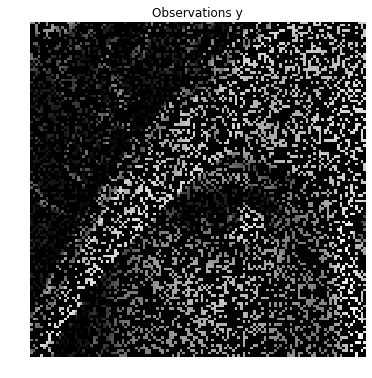## Soft Thresholding in a Basis¶

The soft thresholding operator is at the heart of $\ell^1$ minimization schemes. It can be applied to coefficients $a$, or to an image $f$ in an ortho-basis.

The soft thresholding is a 1-D functional that shrinks the value of coefficients. $$s_T(u)=\max(0,1-T/|u|)u$$

Define a shortcut for this soft thresholding 1-D functional.

In :
SoftThresh = lambda x, T: x*np.maximum(1-T/np.maximum(abs(x), 1e-10*np.ones(np.shape(x))), np.zeros(np.shape(x)))


Display a curve of the 1D soft thresholding.

In :
x = np.linspace(-1, 1, 1000)

plt.figure(figsize=(7,5))
plt.plot(x, SoftThresh(x,.5))
plt.show()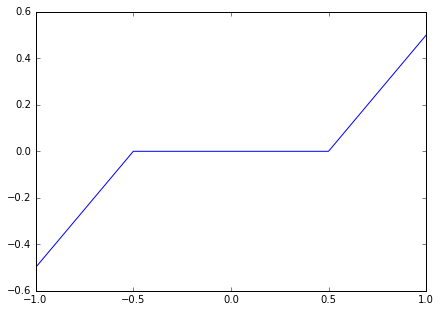Note that the function SoftThresh can also be applied to vector which defines an operator on coefficients: $$S_T(a) = ( s_T(a_m) )_m.$$

In the next section, we use an orthogonal wavelet basis $\Psi$.

We set the parameters of the wavelet transform.

In :
Jmax = np.log2(n)-1
Jmin = (Jmax-3)


Shortcut for $\Psi$ and $\Psi^*$ in the orthogonal case.

In :
from nt_toolbox.perform_wavelet_transf import *

Psi = lambda a: perform_wavelet_transf(a, Jmin, -1, ti=0)
PsiS = lambda f: perform_wavelet_transf(f, Jmin, +1, ti=0)


The soft thresholding opterator in the basis $\Psi$ is defined as $$S_T^\Psi(f) = \sum_m s_T( \langle f,\psi_m \rangle ) \psi_m$$

It thus corresponds to applying the transform $\Psi^*$, thresholding the coefficients using $S_T$ and then undoing the transform using $\Psi$. $$S_T^\Psi(f) = \Psi \circ S_T \circ \Psi^*$$

In :
SoftThreshPsi = lambda f, T: Psi(SoftThresh(PsiS(f), T))


This soft thresholding corresponds to a denoising operator.

In :
plt.figure(figsize=(6,6))
imageplot(clamp(SoftThreshPsi(f0, 0.1)))## Inpainting using Orthogonal Wavelet Sparsity¶

If $\Psi$ is an orthogonal basis, a change of variable shows that the synthesis prior is also an analysis prior, that reads $$f^{\star} \in \text{argmin}_f \: E(f) = \frac{1}{2}\|y-\Phi f\|^2 + \lambda \sum_m \|\langle f,\psi_m \rangle\|.$$

To solve this non-smooth optimization problem, one can use forward-backward splitting, also known as iterative soft thresholding.

It computes a series of images $f^{(\ell)}$ defined as $$f^{(\ell+1)} = S_{\tau\lambda}^{\Psi}( f^{(\ell)} - \tau \Phi^{*} (\Phi f^{(\ell)} - y) )$$

Set up the value of the threshold.

In :
lambd = .03


In our setting, we have $\Phi^* = \Phi$ which is an operator of norm 1.

For $f^{(\ell)}$ to converge to a solution of the problem, the gradient step size should be chosen as $$\tau < \frac{2}{\|\Phi^* \Phi\|} = 2$$

In the following we use: $$\tau = 1$$

Since we use $\tau=1$ and $\Phi = \Phi^* = \text{diag}(1-\Omega)$, the gradient descent step is a projection on the inpainting constraint $$C = \{ f \backslash \forall \Omega(x)=0, f(x)=y(x) \}$$ One thus has $$f - \tau \Phi^{*} (\Phi f - y) = \text{Proj}_C(f)$$

For the sake of simplicity, we define a shortcut for this projection operator.

In :
ProjC = lambda f, Omega: Omega*f + (1-Omega)*y


Each iteration of the forward-backward (iterative thresholding) algorithm thus reads: $$f^{(\ell+1)} = S_{\lambda}^\Psi( \text{Proj}_C(f^{(\ell)}) ).$$

Initialize the iterations.

In :
fSpars = y


In :
fSpars = ProjC(fSpars, Omega)


Second step: denoise the solution by thresholding.

In :
fSpars = SoftThreshPsi(fSpars, lambd)


Exercise 1

Perform the iterative soft thresholding. Monitor the decay of the energy $E$ you are minimizing.

In :
run -i nt_solutions/inverse_5_inpainting_sparsity/exo1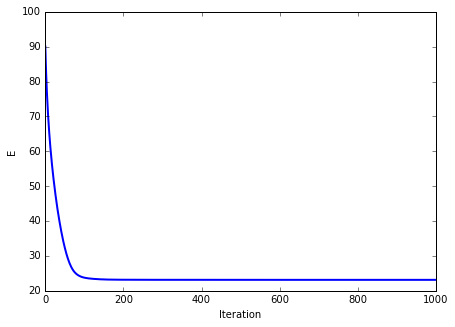In :
## Insert your code here.


Display the result.

In :
plt.figure(figsize=(6,6))
imageplot(clamp(fSpars))Exercise 2

Since there is no noise, one should in theory take $\lambda \rightarrow 0$. To do this, decay the value of $\lambda$ through the iterations.

In :
run -i nt_solutions/inverse_5_inpainting_sparsity/exo2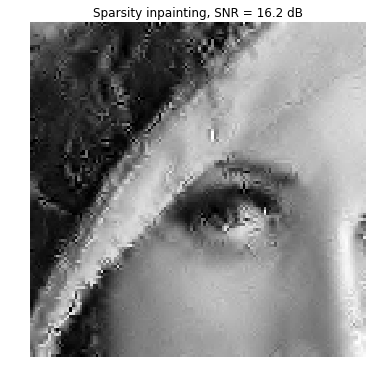In :
## Insert your code here.


## Inpainting using Translation Invariant Wavelet Sparsity¶

Orthogonal sparsity performs a poor regularization because of the lack of translation invariance. This regularization is enhanced by considering $\Psi$ as a redundant tight frame of translation invariant wavelets.

One thus looks for optimal coefficients $a^\star$ that solves $$a^{\star} \in \text{argmin}_a \: E(a) = \frac{1}{2}\|y-\Phi \Psi a\|^2 + \lambda J(a)$$

Important: The operator $\Psi^*$ is the forward translation invariant wavelet transform. It computes the inner product with the unit norm wavelet atoms: $$(\Psi^* f)_m = \langle f,\psi_m \rangle \quad \text{with} \quad \|\psi_m\|=1.$$

The reconstruction operator $\Xi$ satisfies $\Xi \Psi^* f = f$, and is the pseudo inverse of the analysis operator $\Xi = (\Psi^*)^+$.

For our algorithm, we will need to use $\Psi$ and not $\Xi$. Lukily, for the wavelet transform, one has $$\Xi = \Psi \text{diag(U)} f$$ where $U_m$ account for the redundancy of the scale of the atom $\psi_m$.

Compute the scaling factor (inverse of the redundancy).

In :
J = Jmax-Jmin + 1
u = np.hstack(([4**(-J)], 4**(-np.floor(np.arange(J + 2./3,1,-1./3)))))
U = np.transpose(np.tile(u, (n,n,1)),(2,0,1))


Choose a value of the regularization parameter.

In :
lambd = .01


Shortcut for the wavelet transform and the reconstruction.

Important: Scilab users have to create files |Xi.m|, |PsiS.m| and |Psi.m| to implement this function.

In :
Xi = lambda a: perform_wavelet_transf(a, Jmin, -1, ti=1)
PsiS = lambda f: perform_wavelet_transf(f, Jmin, + 1, ti=1)
Psi = lambda a: Xi(a/U)


The forward-backward algorithm now compute a series of wavelet coefficients $a^{(\ell)}$ computed as $$a^{(\ell+1)} = S_{\tau\lambda}( a^{(\ell)} + \Psi^*\Phi( y - \Phi\Psi a^{(\ell)} ) ).$$

The soft thresholding is defined as: $$\forall m, \quad S_T(a)_m = \max(0, 1-T/\|a_m\|)a_m.$$

The step size should satisfy: $$\tau < \frac{2}{\|\Psi\Phi \|} \leq 2 \min( u ).$$

In :
tau = 1.9*np.min(u)


Initialize the wavelet coefficients with those of the previous reconstruction.

In :
a = U*PsiS(fSpars)


In :
fTI = Psi(a)
a = a + tau*PsiS(Phi(y-Phi(fTI, Omega), Omega))


Soft threshold.

In :
a = SoftThresh(a, lambd*tau)


Exercise 3

Perform the iterative soft thresholding. Monitor the decay of the energy $E$.

In :
run -i nt_solutions/inverse_5_inpainting_sparsity/exo3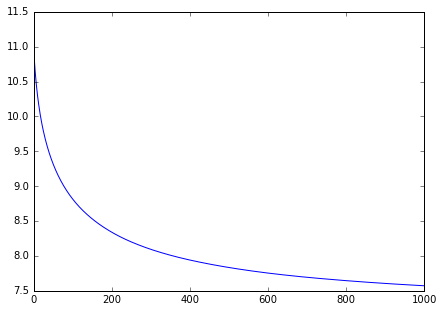In :
## Insert your code here.


Perform the reconstruction.

In :
fTI = Psi(a)


Display the result.

In :
plt.figure(figsize=(6,6))
imageplot(clamp(fTI))Exercise 4

Perform the iteration with a decaying value of $\lambda$

In :
run -i nt_solutions/inverse_5_inpainting_sparsity/exo4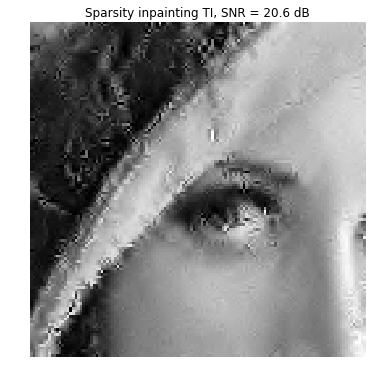In :
## Insert your code here.


## Inpainting using Iterative Hard Thresholding¶

To improve the sparsity of the solution, it is possible to replace the soft thresholding by a hard threshdoling. In this case, the resulting algorihtm does not perform anymore a variational minimization of an energy.

The hard thresholding is defined as $h_T(x)=0$ if $-T < x < T$ and $h_T(x)=x$ otherwise. It thus defines a thresholding operator of wavelet coefficients as $H_T(a)_m = h_T(a_m)$.

Define a shortcut for this vectorialized hard thresholding

Important: Scilab users have to create a file |HardThresh.m| to implement this function.

In :
HardThresh = lambda x, t: x*(abs(x) > t)


Display a curve of the 1-D Hard thresholding.

In :
x = np.linspace(-1, 1, 1000)

plt.figure(figsize=(7,5))
plt.plot(x, HardThresh(x, .5))
plt.show()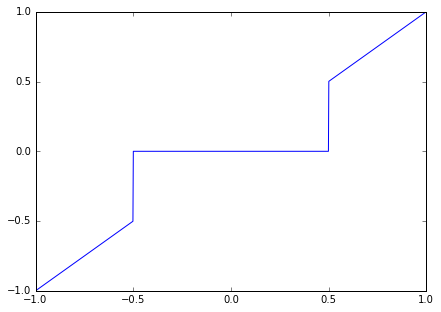The hard thresholding in the translation invariant wavelet basis $\Psi$ reads $$H_T^\Psi(f) = \Xi \circ H_T \circ \Psi^* (f)$$ where $\Xi = (\Phi^*)^+$ is the reconstruction operator.

We follow the MCA paradigm of Jean-Luc Starck, that alternates between a gradient descent step and a hard thresholding denoising, using a decaying threshold. $$f^{(\ell+1)} = H_{\tau\lambda_\ell}^\Psi( f^{(\ell)} - \tau \Phi^*(\Phi f^{(\ell)} - y) ).$$

Number of iterations.

In :
niter = 500


List of thresholds. One must start by a large enough initial threshold.

In :
lambda_list = np.linspace(1, 0, niter)


Initialization.

In :
fHard = y


In :
fHard = ProjC(fHard, Omega)


Hard threshold (here $\lambda=\lambda_0$) is used).

In :
fHard = Xi(HardThresh(PsiS(fHard), tau*lambda_list))


Exercise 5

Perform the iteration with a decaying value of $\lambda$

In :
run -i nt_solutions/inverse_5_inpainting_sparsity/exo5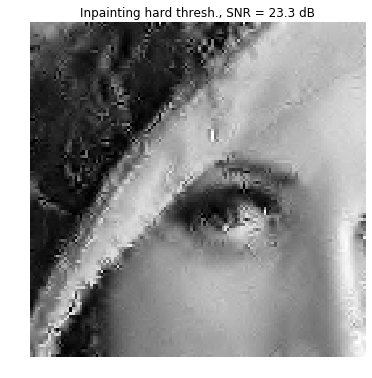In :
## Insert your code here.